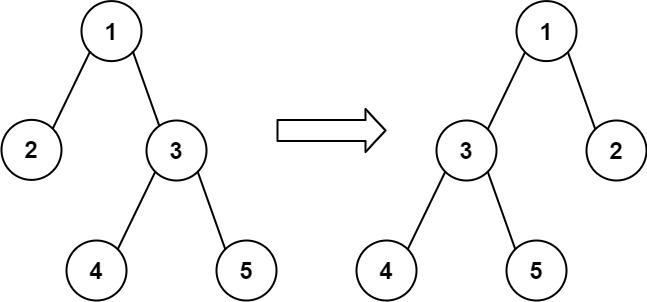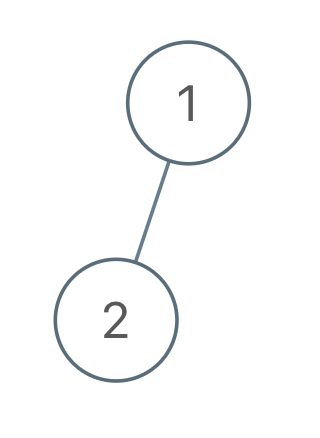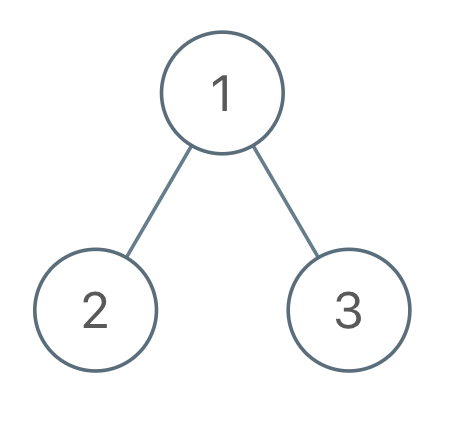# 翻转二叉树以匹配先序遍历

## 971. 翻转二叉树以匹配先序遍历 (Medium)```输入：root = [1,2], voyage = [2,1]

``````输入：root = [1,2,3], voyage = [1,3,2]```输入：root = [1,2,3], voyage = [1,2,3]

```

• 树中的节点数目为 `n`
• `n == voyage.length`
• `1 <= n <= 100`
• `1 <= Node.val, voyage[i] <= n`
• 树中的所有值 互不相同
• `voyage` 中的所有值 互不相同

[] [深度优先搜索]